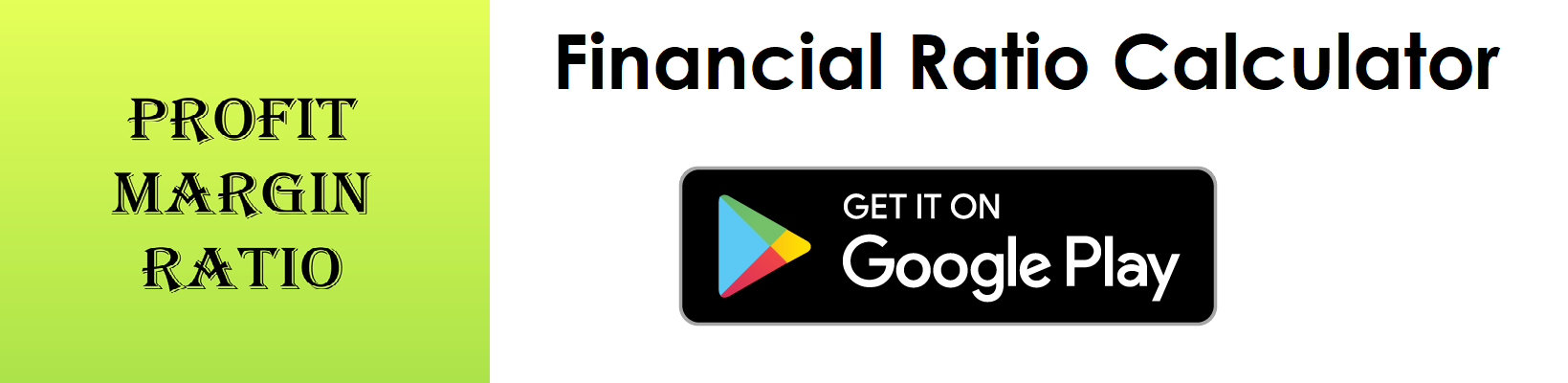# Profit Margin Ratio Calculator

Key in the Earnings and Net Income Values from your Income Statement to the respective fields given below and then click Calculate to get the desired result.

##### Profit Margin Ratio Calculator - Glossary:
Using our profit margin ratio calculator, helps to compare, measure, understand the overall health of the business.

##### Profit Margin:
Shows the proportion of Sales to Net Income. In simple words, it is expressed in terms of percentage. It helps to measure how much net profit was made from its earnings.

##### How to calculate?
Formula: Profit Margin = Net Income/Net Sales.
This is an income statement component, the values are commonly stated against Net Income and Net Sales (Earnings).

##### Net Sales:
Total sales less returns and bad debts.

##### Net Income:
Profit left over after deducting all its expenses (cost of goods, operational expenses, interest cost, depreciation cost, taxes and if any dividend paid).

##### Example:
Profit margin for a company with a net sales income of \$100,000 and net income of \$10,000 is 10%. It means the company has a net income of \$0.10 for each dollar it generated, or we can say that, company converted 10% of its sales into profits.

# Download our Mobile Apps - today.